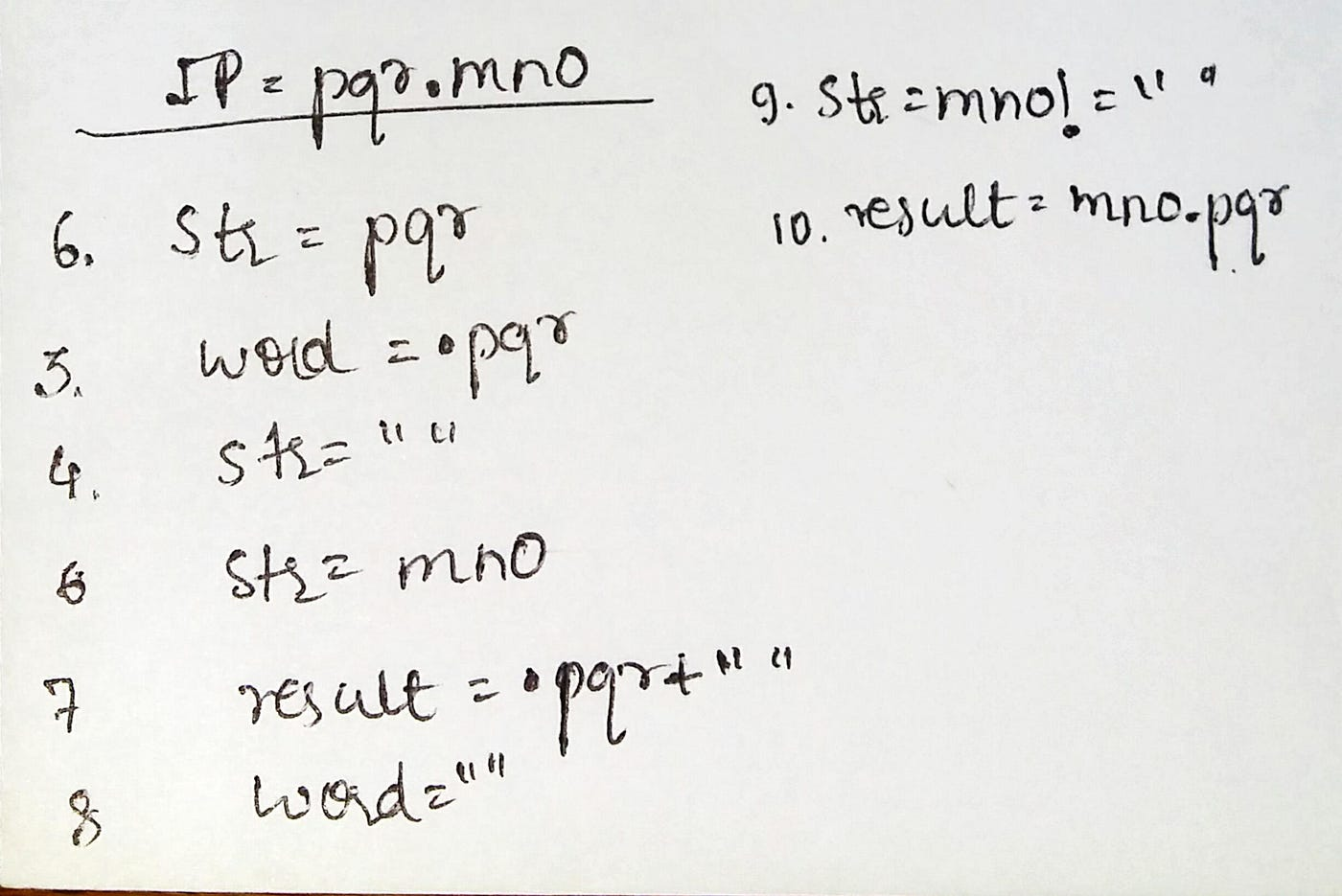# Reversing Words

## String Manipulation Example

Given a String S, reverse the string without reversing its individual words. Words are separated by dots.

Example 1:

`Input:S = i.like.this.program.very.muchOutput: much.very.program.this.like.iExplanation: After reversing the wholestring(not individual words), the inputstring becomesmuch.very.program.this.like.i`

Example 2:

`Input:S = pqr.mnoOutput: mno.pqrExplanation: After reversing the wholestring , the input string becomesmno.pqr`

You don't need to read input or print anything. Complete the function reverseWords() which takes string S as input parameter and returns a string containing the words in reversed order. Each word in the returning string should also be separated by ‘.’

Expected Time Complexity: O(|S|)
Expected Auxiliary Space: O(|S|)

Constraints:
1 <= |S| <= 2000

# Approach :

1. Having O(|s|) space complexity & O(|s|) time complexity where |s| is the length of the given string
`class Solution{    public:    //Function to reverse words in a given string.    string reverseWords(string s)     {         string str="";        string result = "";        string word;        1      for(int i=0;s[i]!='\0';i++)        {2           if(s[i]=='.')            {3               word = s[i]+str;4               str="";            }5           else            {6               str+=s[i];            }7       result = word+result;8       word="";        }9       if(str!="")10      result = str+result;11      return result;    } };Below I will dry run an input to help you understand better.`You can infer from the above code using the numbers which have been marked and can easily understand how this question has been solved.

I am simply adding the dots before the word, like for eg above I made the word as .pqr and then appended it with the later word. So using this logic it becomes easy to get the desired output.